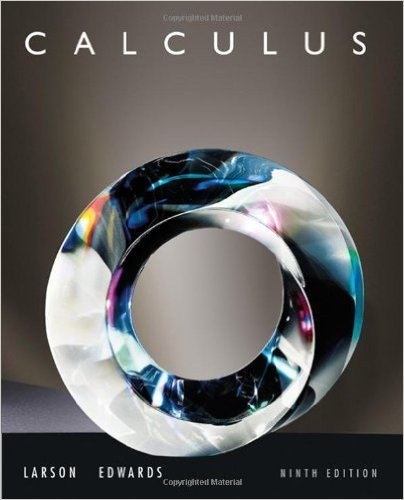×
×

# Answer: In Exercises 14, (a) find the domain of and (b) determine the values (if any) ofISBN: 9780547167022 357

## Solution for problem 2 Chapter 12

Calculus | 9th Edition

• Textbook Solutions
• 2901 Step-by-step solutions solved by professors and subject experts
• Get 24/7 help from StudySoup virtual teaching assistantsCalculus | 9th Edition

4 5 0 358 Reviews
28
5
Problem 2

In Exercises 14, (a) find the domain of and (b) determine the values (if any) of for which the function is continuous.

Step-by-Step Solution:
Step 1 of 3

,"2' f") rj. --' uo'[").] I _12. Z-/ .(fl+ elxq l =x9 ottl ,Y \-'lo(rt- { Lul.II...

Step 2 of 3

Step 3 of 3

##### ISBN: 9780547167022

This full solution covers the following key subjects: . This expansive textbook survival guide covers 125 chapters, and 10706 solutions. Since the solution to 2 from 12 chapter was answered, more than 222 students have viewed the full step-by-step answer. The answer to “In Exercises 14, (a) find the domain of and (b) determine the values (if any) of for which the function is continuous.” is broken down into a number of easy to follow steps, and 22 words. Calculus was written by and is associated to the ISBN: 9780547167022. This textbook survival guide was created for the textbook: Calculus , edition: 9. The full step-by-step solution to problem: 2 from chapter: 12 was answered by , our top Calculus solution expert on 03/08/18, 08:41PM.

Unlock Textbook Solution